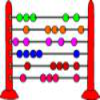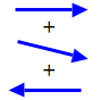#### You may also like### Fibonacci's Three Wishes 1

First of two articles about Fibonacci, written for students.### Arrow Arithmetic 1

The first part of an investigation into how to represent numbers using geometric transformations that ultimately leads us to discover numbers not on the number line.### A Story about Absolutely Nothing

This article for the young and old talks about the origins of our number system and the important role zero has to play in it.

# Balance Power

##### Age 11 to 18Challenge Level

Why do this problem?
Learners can discover the base two number system for themselves and gain experience of working with powers of 2 and 3. The problem can be used to reinforce understanding of place value.

The generalisation to the problem which allows weights in both pans leads naturally to representing numbers in base 3.

Possible approach
As a class, work together through weighing 1, 2, 3, 4, 5 and 6 units with the fewest weights possible and then ask the learners to continue the process.

Key questions
Weights in one pan only
What weights are needed to weigh 1, 2, 3,....15 units?

What pattern do you notice in the weights used? Can you explain it?

When you include another weight in the set of weights used how many objects can now be weighed?

How many weights are needed to weigh objects of 1, 2, 3 ... 100 units?

How many weights are needed to weigh 1 to 1000 units or 1 to n units?

Weights in both pans
What weights are needed to weigh 1, 2, 3,....13 units?

What pattern do you notice in the weights used? Can you explain it?

When you include another weight in the set of weights used how many objects can now be weighed?

How many weights are needed to weigh objects of 1, 2, 3 ... 100 units?

How many weights are needed to weigh 1 to 1000 units or 1 to n units?

Possible extension
Discuss with the class the idea that, using both pans, the weights are used either as +1 times the weight (in the opposite pan to the object), 0 times the weight (not used at all), or -1 times the weight (in the same pan as the object). We are using a numbering system

$$a_0 + a_1x + a_2x^2 + a_3x^3 + ...$$ where the coefficients $a_0, a_1$ etc. are all 1, 0 or -1 and $x=3$. By a (reversible) 'shift' this is changed to the base 3 number system.

All integers can be written in base 3 with digits 0, 1 and 2, that is as $$b_0 + b_1x + b_2x^2 + b_3x^3 + ...$$ where the coefficients $b_0, b_1$ etc. are all 0, 1 or 2 and $x=3$. This explains why all objects weighing an integer number of units can be weighed with a set of weights that are all powers of 3.

You may find a spreadsheet tool useful.